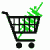July 31, 2019

# How to measure DC electrical current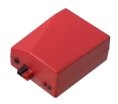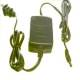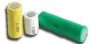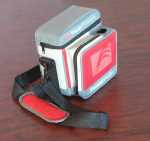Battery capacity tester for small and tiny cells Chargers Batteries and Packs 7.5-22 AH portable power stations Inexpensive Battery Spot Welder More Engineering Resources

## Introduction: the plumbing analogy

You might have heard this before. Electricity and plumbing are analogous. In one case you have electrons flowing in a wire, and in the other case you have water flowing in a pipe. The thing that makes water move in a pipe is pressure. The thing that makes electrons move in a wire is voltage. The amount of water moved in a pipe is measured in gallons. The number of electrons moved, or the amount of charge moved through a wire is measured in coulombs (a coulomb is just a fixed quantity of electrons, like a dozen, but bigger).

Here is a table showing the analogy
 Concept Plumbing Electricity Comments Motivating "force" Pressure Voltage Quantity Gallons Coulombs 6.24 x 1018 electrons per coulomb Rate of flow Gallons per minute Amps 1 Amp is one Coulomb per second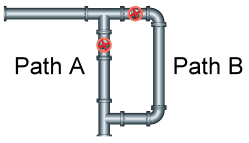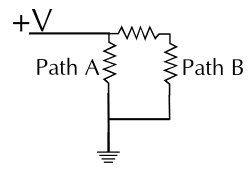Plumbing version of the basic circuit Electrical version of the basic circuit

Now the question is how do you measure the rate of flow, or current in one of the paths?

Pressure or voltage is relatively easy. In the plumbing case you just drill a hole in the pipe and add a pressure gauge. In the electrical case you connect a volt meter between the point of interest and the ground.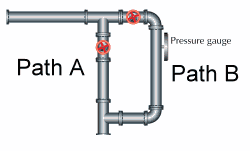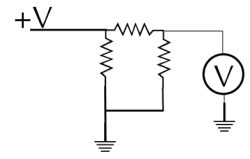Measuring pressure, just drill and tap a hole in the pipe and screw in a pressure gauge Measuring the voltage at any point in the circuit

To measure the flow in the pipe you have to cut the pipe and insert a flow gauge. To measure the current you have to cut the circuit and install an ammeter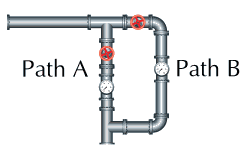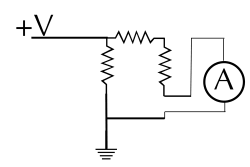Flow meters must be inserted into the flowby cutting the pipe. Ammeters must also be inserted into the flow of the current by cutting the circuit

## How is amperage actually measured

There are two properties of moving charges that are useful for measuring current
1. Electric current flowing through a resistance results in a voltage drop according to Ohm's Law
2. Electric current will always be associated with a magnetic field.

Let's first look at the use of Ohm's law. Assuming that voltage is easy to measure, what you do is introduce a known fixed resistance into the circuit. Then the voltage measured across the resistor is proportional to the current flowing through it. To make a good measurement the resistor must be precise in value and stable (not change resistance with temperature, pressure, illumination, etc.) And in order to keep from disturbing the circuit too much it must also be low in value. This resistor is often erroneously known as a shunt resistor, but a better term for it is sense resistor. According to Ohm's law V = I·R if the sense resistor is 1 ohm, then the voltage across it will be 1 volt per amp. Here is a practical use of this technique.

Here is a practical application of current measurement. It has application to power supply design: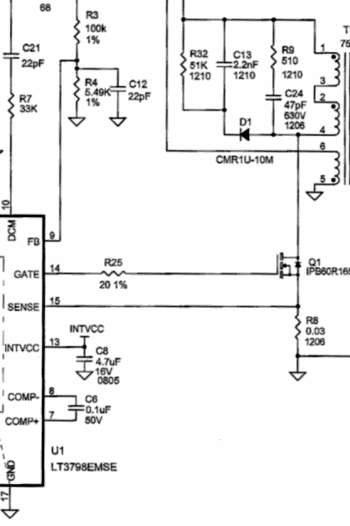Here the engineer is trying to control a power circuit. Q1 is energizing the transformer T with pulses, but he needs to know how much current is flowing through the transistor during the pulse. He uses resistor R8, which is only 0.03 ohms as a sense resistor. For every amp flowing through the resistor 0.03V, or 30mV appears across it, which is detected by the sense input of the PWM controller, Pin 15. The PWM controller uses this data to control the current, voltage or power output of the power supply, depending on the rest of the circuit.

One of the earliest ways to detect electric current was a few wraps of wire around a compass. D'Arsenval extended this idea to make an amperage measurement device (ammeter) by putting a coil inside magnetic poles with bearings and watch escapement springs. This is the way that analog meters work today.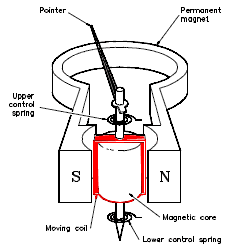Another way to make use of the magnetic field is with a clamp on DC ammeter. These use the Hall effect to measure very weak magnetic fields created by electricity in a wire. These are fairly recent inventions, and are extremely convenient, since in this case you do not have to cut the wire to insert a traditional ammeter.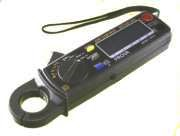A clamp-on DC reading ammeter from PowerStreamThank you for considering PowerStream

 PowerStream Technology 1163 South 1680 West Orem, Utah 84058 USA Phone: 801-764-9060 Fax: 801-764-9061 © Copyright 2000, 2002, 2003, 2004, 2005, 2006, 2008,2009, 2010, 2011, 2012, 2013, 2014, 2015, 2016, 2017, 2018, 2019, 2020, 2021, 2022 Lund Instrument Engineering, Inc. All rights reserved. Google Author Verification